# Object Detection Analysis of RefComplex Images

## 11 July 2014

### LOAD PACKAGES

``````rm(list=ls())
library('entropy')
library('reshape')
library('ggplot2')
``````

## Analysis 1: Entropy of objects

Merge object weight files into single data frame

``````#setwd("/Documents/GRADUATE_SCHOOL/Projects/ref_complex/obj_feature_complexity/objs/")  set working directory and read in data
#f = list.files() # get all obj weight files

# merge obj weight files into single data frame
#for (i in 1:length(f)) {
# d <- read.csv(f[i], header = FALSE)
# names(d) = c("obj", paste('rc',d[1,3], sep = ""))
# d[,3] <- NULL
# if (i != 1) {
#  weights = merge(weights, d ,"obj")
#} else {
#  weights = d
#}
#}
#write.csv(weights, "object_weights.csv")
``````

Load object weights file (Each row a feature, each column an RC object)

``````weights = read.csv('/Documents/GRADUATE_SCHOOL/Projects/ref_complex/obj_feature_complexity/objs/object_weights.csv')
weights = weights[,-c(1,2)] # get rid of extra columns
dim(weights)
``````
``````##  1000   62
``````

Get object entropy and add object column

``````#entropy
e = melt(sapply(weights,function(x) entropy(x, method = "ML")))

#object column
names(e) = 'entropy'
e\$o = row.names(e)
e = e[-1,]
e\$obj = as.factor(as.numeric(as.character(sapply(strsplit(e\$o,'rc'), function(x) strsplit(x,'rc') ))))
e\$o <- NULL

# get rid of anchors
e = e[e\$obj !=  "61",]
e = e[e\$obj !=  "62",]
``````

Merge in norms

``````c_norms <- read.csv("/Documents/GRADUATE_SCHOOL/Projects/ref_complex/Experiment_9_norm/complicated1AND2_norms.csv")
index <- match(e\$obj, c_norms\$ratingNum )
e\$c.norms <- c_norms\$value[index]

rt_norms <- read.csv("/Documents/GRADUATE_SCHOOL/Projects/ref_complex/Experiment_30/Analysis/rt_norms.csv")
index <- match(e\$obj,  rt_norms\$Answer.train_image  )
e\$log.rt.norms <- rt_norms\$log.rt [index]
``````

Look at data distributions

``````summary(e)
``````
``````##     entropy           obj        c.norms       log.rt.norms
##  Min.   :0.363   2      : 1   Min.   :0.145   Min.   :7.16
##  1st Qu.:2.505   3      : 1   1st Qu.:0.332   1st Qu.:7.26
##  Median :3.097   4      : 1   Median :0.441   Median :7.31
##  Mean   :2.890   5      : 1   Mean   :0.465   Mean   :7.32
##  3rd Qu.:3.534   6      : 1   3rd Qu.:0.607   3rd Qu.:7.37
##  Max.   :4.282   7      : 1   Max.   :0.889   Max.   :7.55
##                  (Other):53
``````
``````hist(e\$entropy)
``````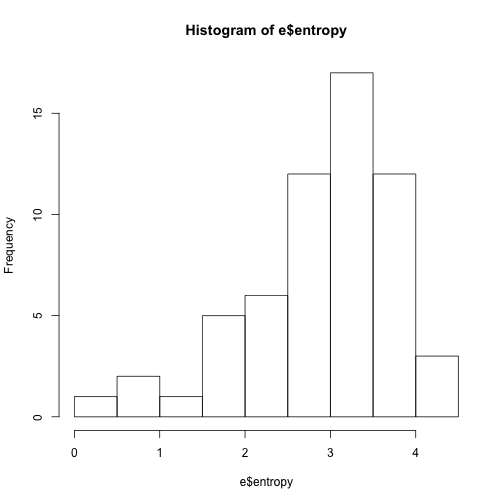``````hist(e\$c.norms)
``````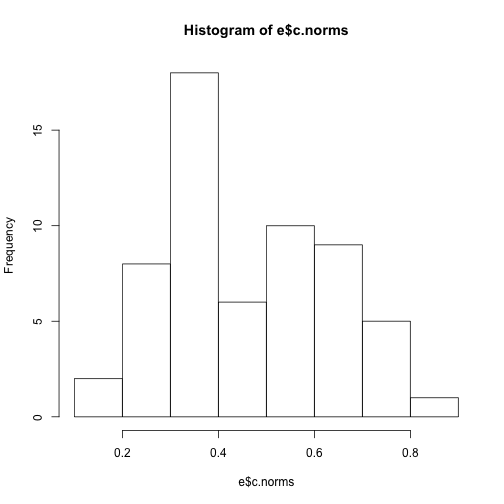``````hist(e\$log.rt.norms)
``````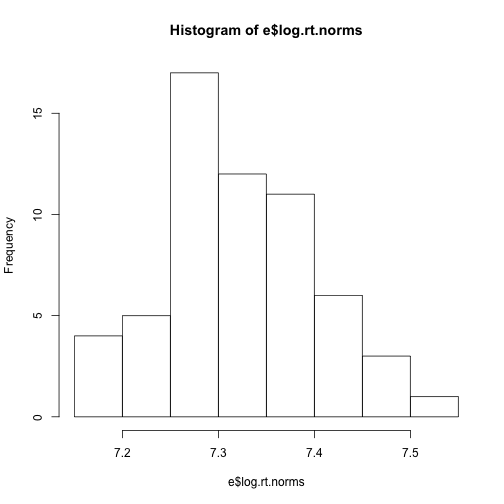Look at relationships

``````qplot(e\$entropy, e\$log.rt.norms)+ geom_smooth(method = lm)
``````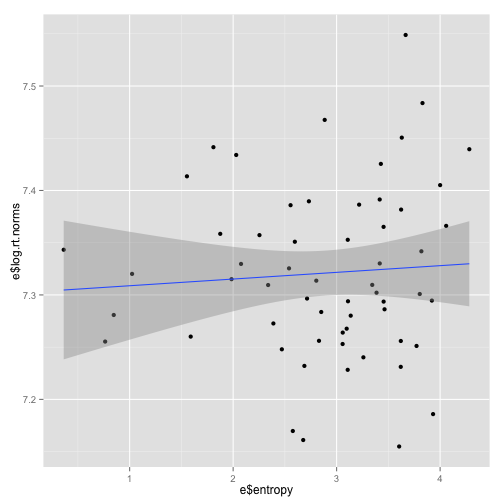``````qplot(e\$entropy, e\$c.norms)+ geom_smooth(method = lm)
``````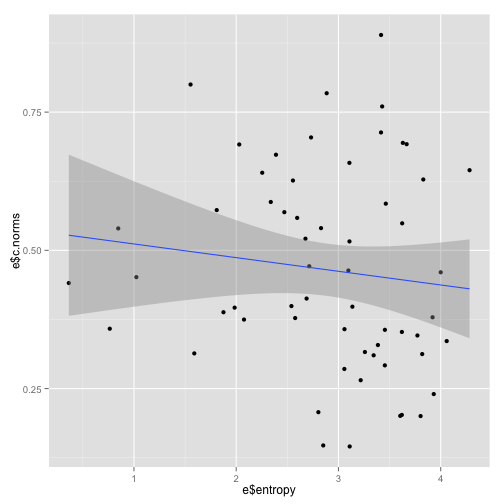Correlations

``````cor.test(e\$entropy, e\$log.rt.norms)
``````
``````##
##  Pearson's product-moment correlation
##
## data:  e\$entropy and e\$log.rt.norms
## t = 0.5162, df = 57, p-value = 0.6077
## alternative hypothesis: true correlation is not equal to 0
## 95 percent confidence interval:
##  -0.1912  0.3187
## sample estimates:
##     cor
## 0.06821
``````
``````cor.test(e\$entropy, e\$c.norms)
``````
``````##
##  Pearson's product-moment correlation
##
## data:  e\$entropy and e\$c.norms
## t = -0.907, df = 57, p-value = 0.3682
## alternative hypothesis: true correlation is not equal to 0
## 95 percent confidence interval:
##  -0.3642  0.1411
## sample estimates:
##     cor
## -0.1193
``````

—> NO relationship between object entropy and norms

## Analysis 2: Object matches names

Read in label data (look at first labels only)

``````labels <- read.csv('/Documents/GRADUATE_SCHOOL/Projects/ref_complex/obj_feature_complexity/object_labels.csv', header = FALSE)
weights\$label = as.character(labels\$V1) #look at first label only
weights\$label_length = nchar(weights\$label)
``````

Figure out which object matches to consider. Either top n matches or whichever matches with weights greater than v. Here, I calculated v by finding 2sd above mean of all weights.

``````#len = melt(sapply(weights,function(x)  mean(weights[order(x)[990:1000], 'label_length']) ))
# figure out cut off for weights
m = melt(weights[1:62])
``````
``````## Using  as id variables
``````
``````v = mean(m\$value) + (2*sd(m\$value))
hist(summary(m[which(m\$value > v),"variable"]), main = "Distribution of number of objects matches for each of the RC objects")
``````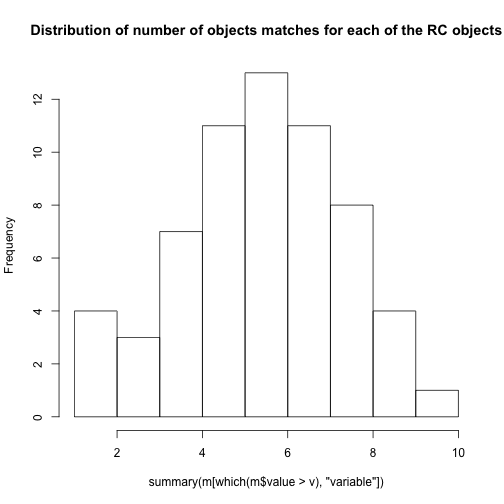``````# Get mean length of each of the matches, for each RC objects
len = melt(sapply(weights,function(x)  mean(weights[x > v, 'label_length']) ))
``````

Get object column

``````names(len) = 'len'
len\$o = row.names(len)
len = len[-c(63,64),]
len\$obj = as.factor(as.numeric(as.character(sapply(strsplit(len\$o,'rc'), function(x) strsplit(x,'rc') ))))
len\$o <- NULL
``````

merge with entropy dataframe

``````index <- match(e\$obj, len\$obj )
e\$len <- len\$len[index]
``````

Look at data

``````qplot(e\$len, e\$log.rt.norms)  + geom_smooth(method = lm)
``````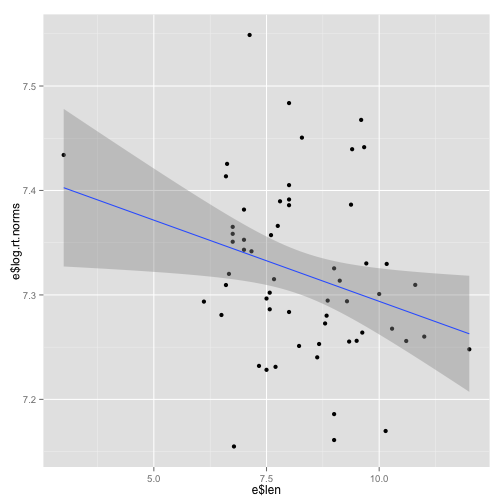``````qplot(e\$len, e\$c.norms) + geom_smooth(method = lm)
``````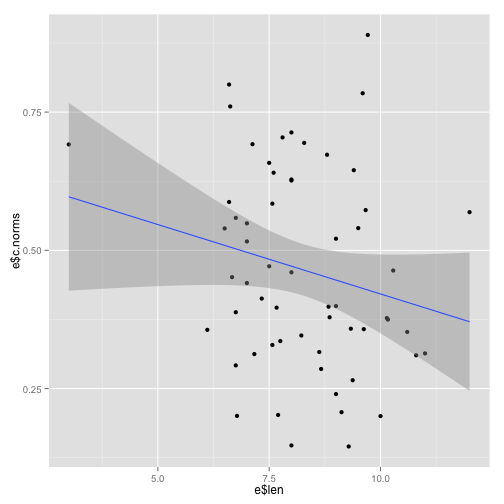Look at at correlations

``````cor.test(e\$len, e\$log.rt.norms)
``````
``````##
##  Pearson's product-moment correlation
##
## data:  e\$len and e\$log.rt.norms
## t = -2.256, df = 57, p-value = 0.0279
## alternative hypothesis: true correlation is not equal to 0
## 95 percent confidence interval:
##  -0.50537 -0.03266
## sample estimates:
##     cor
## -0.2864
``````
``````cor.test(e\$len, e\$c.norms)
``````
``````##
##  Pearson's product-moment correlation
##
## data:  e\$len and e\$c.norms
## t = -1.619, df = 57, p-value = 0.111
## alternative hypothesis: true correlation is not equal to 0
## 95 percent confidence interval:
##  -0.44200  0.04907
## sample estimates:
##     cor
## -0.2096
``````

—> NEGATIVE relationship between mean object match name length and complexity (i.e. more complex object have shorter mean label length)

## Analysis 3: Entropy of features

Read in feature weights file (Each row is a feature and each column is a RC object)

``````fs <- read.csv("/Documents/GRADUATE_SCHOOL/Projects/ref_complex/obj_feature_complexity/features/feature_weights_normalized.csv", header = TRUE)
dim(fs)
``````
``````##  4096   60
``````

Get entropy. (I'm not really sure what the best method for estimating entropy here is….)

``````ef = melt(sapply(fs,function(x) entropy(x, method = "ML")))

#Add obj column
names(ef) = 'entropy'
ef\$o = row.names(ef)
ef\$obj = as.factor(as.numeric(as.character(sapply(strsplit(ef\$o,'X'), function(x) strsplit(x,'rc') ))))
ef\$o <- NULL
``````

Merge in norms

``````c_norms <- read.csv("/Documents/GRADUATE_SCHOOL/Projects/ref_complex/Experiment_9_norm/complicated1AND2_norms.csv")
index <- match(ef\$obj, c_norms\$ratingNum )
ef\$c.norms <- c_norms\$value[index]

rt_norms <- read.csv("/Documents/GRADUATE_SCHOOL/Projects/ref_complex/Experiment_30/Analysis/rt_norms.csv")
index <- match(ef\$obj,  rt_norms\$Answer.train_image  )
ef\$log.rt.norms <- rt_norms\$log.rt [index]
``````

Look at distribtions and correlations.

``````summary(ef)
``````
``````##     entropy          obj        c.norms       log.rt.norms
##  Min.   :6.16   1      : 1   Min.   :0.145   Min.   :7.16
##  1st Qu.:6.46   2      : 1   1st Qu.:0.334   1st Qu.:7.26
##  Median :6.58   3      : 1   Median :0.446   Median :7.31
##  Mean   :6.59   4      : 1   Mean   :0.465   Mean   :7.32
##  3rd Qu.:6.72   5      : 1   3rd Qu.:0.597   3rd Qu.:7.37
##  Max.   :6.96   6      : 1   Max.   :0.889   Max.   :7.55
##                 (Other):54
``````
``````hist(ef\$entropy)
``````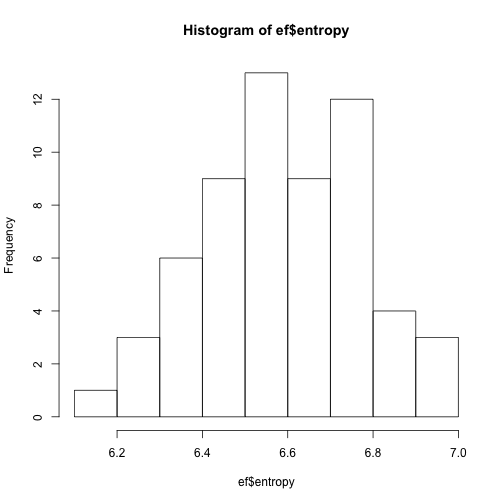``````qplot(ef\$entropy, ef\$log.rt.norms)+ geom_smooth(method = lm)
``````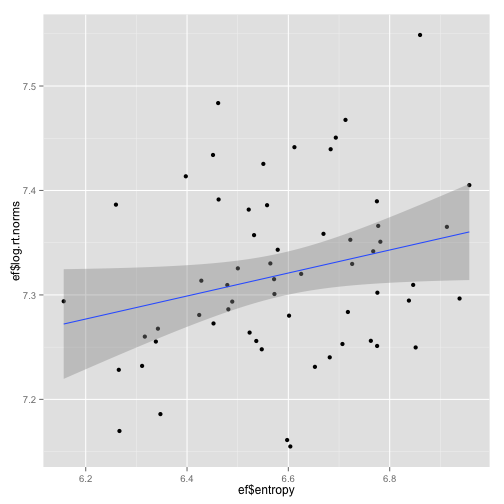``````qplot(ef\$entropy, ef\$c.norms)+ geom_smooth(method = lm)
``````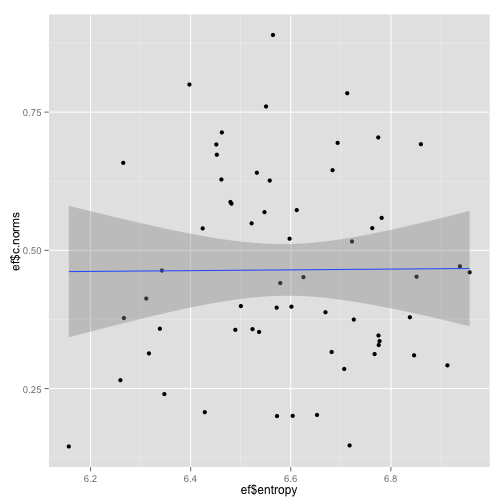Look at correlations

``````cor.test(ef\$entropy, ef\$log.rt.norms)
``````
``````##
##  Pearson's product-moment correlation
##
## data:  ef\$entropy and ef\$log.rt.norms
## t = 1.973, df = 58, p-value = 0.05326
## alternative hypothesis: true correlation is not equal to 0
## 95 percent confidence interval:
##  -0.003343  0.474502
## sample estimates:
##    cor
## 0.2508
``````

—> Feature entropy weakly correlated with RT norms